Скачать презентацию Chapter 10 Standard Costing and Analysis of Direct

f0e80e8a858f449485a835394ff4baef.ppt

• Количество слайдов: 61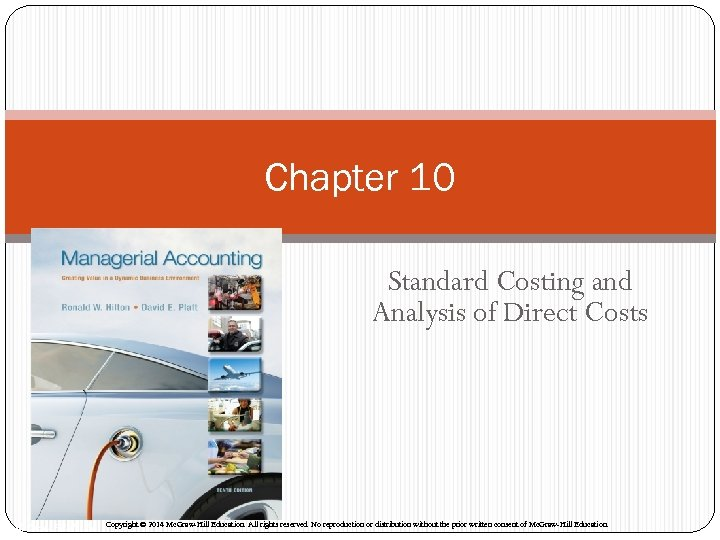Chapter 10 Standard Costing and Analysis of Direct Costs Mc. Graw-Hill/Irwin Copyright © 2014 Mc. Graw-Hill Education. All rights reserved. No reproduction or distribution without the prior written consent of Mc. Graw-Hill Education.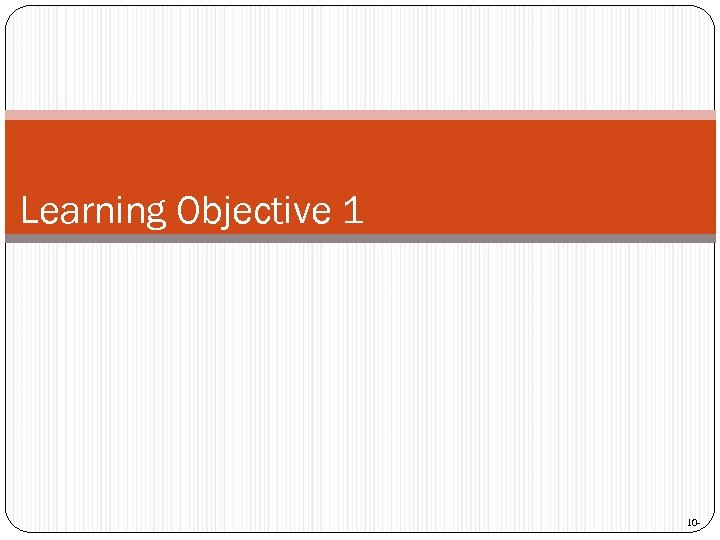Learning Objective 1 10 -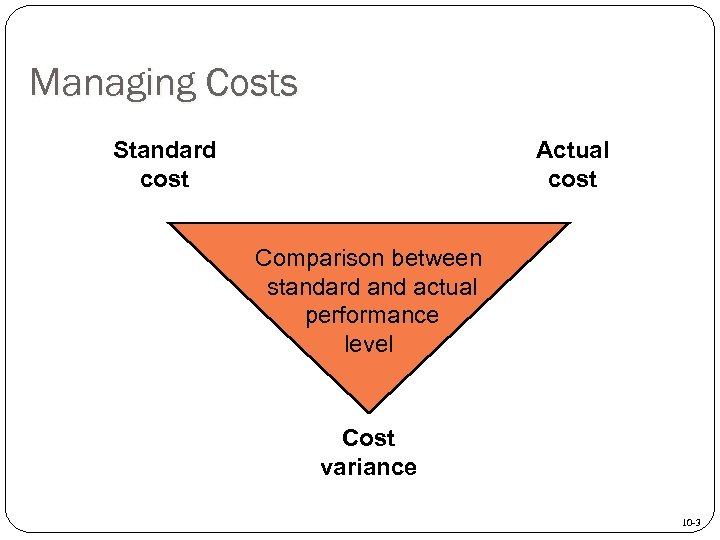Managing Costs Standard cost Actual cost Comparison between standard and actual performance level Cost variance 10 -3Management by Exception Amount Managers focus on quantities and costs that exceed standards, a practice known as management by exception. . Standard Direct Labor Direct Material Type of Product Cost 10 -4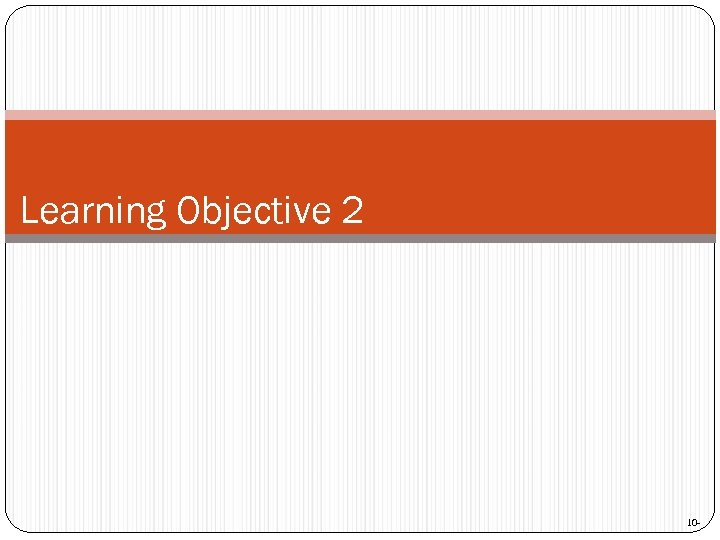Learning Objective 2 10 -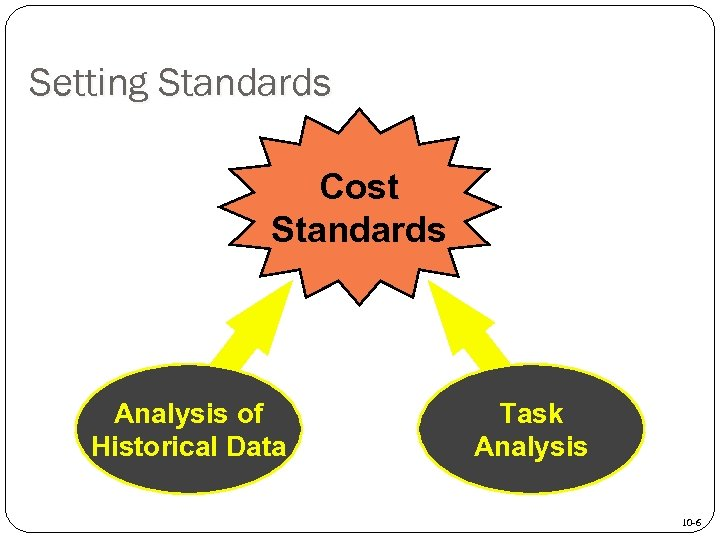Setting Standards Cost Standards Analysis of Historical Data Task Analysis 10 -6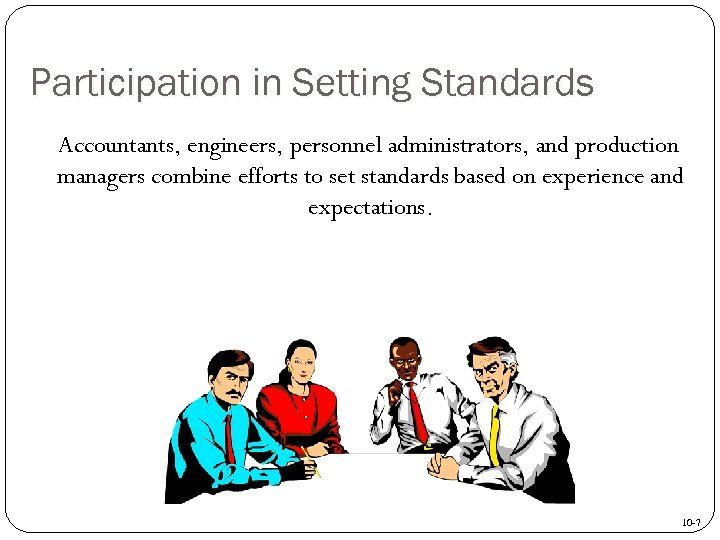Participation in Setting Standards Accountants, engineers, personnel administrators, and production managers combine efforts to set standards based on experience and expectations. 10 -7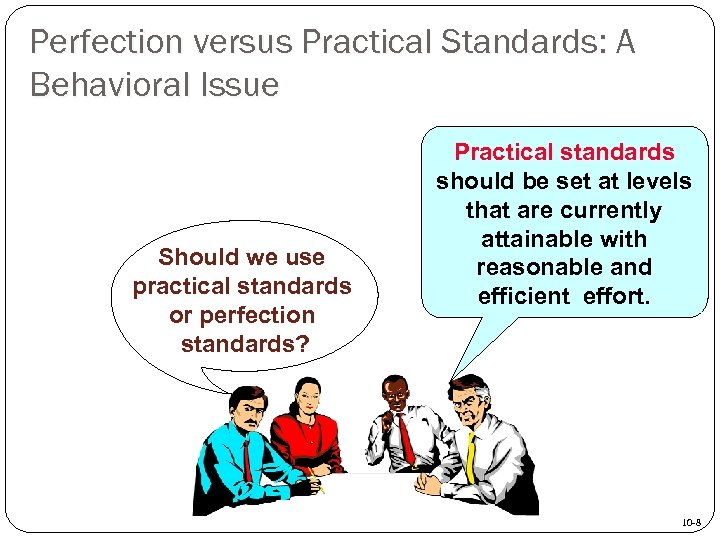Perfection versus Practical Standards: A Behavioral Issue Should we use practical standards or perfection standards? Practical standards should be set at levels that are currently attainable with reasonable and efficient effort. 10 -8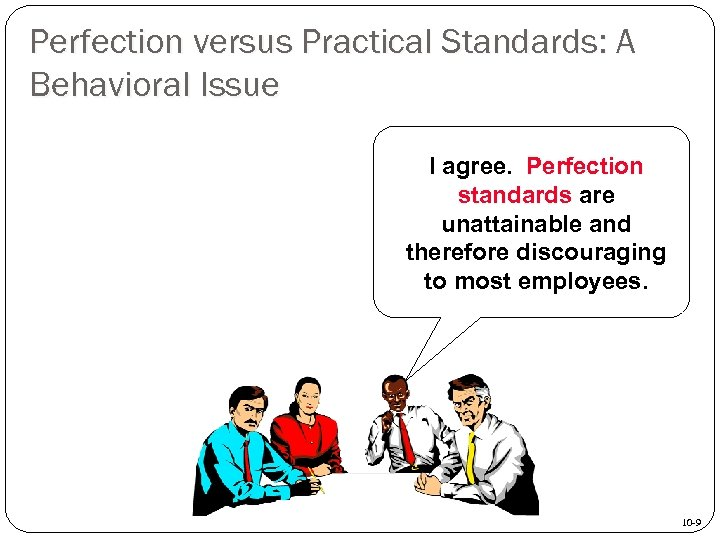Perfection versus Practical Standards: A Behavioral Issue I agree. Perfection standards are unattainable and therefore discouraging to most employees. 10 -9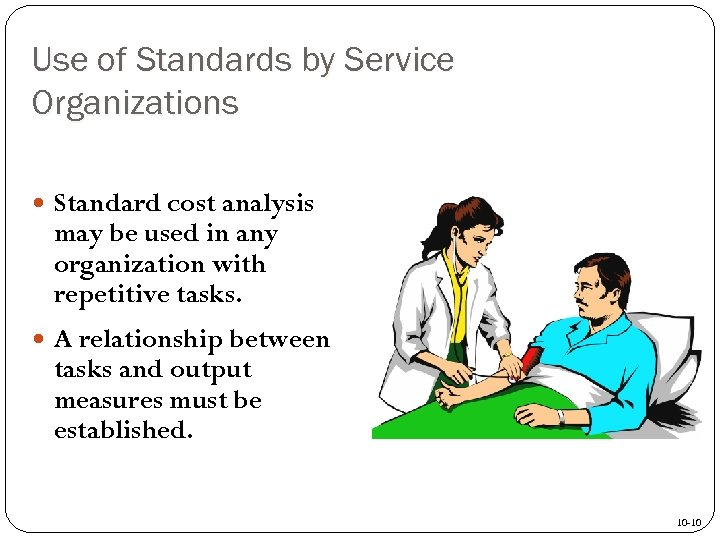Use of Standards by Service Organizations Standard cost analysis may be used in any organization with repetitive tasks. A relationship between tasks and output measures must be established. 10 -10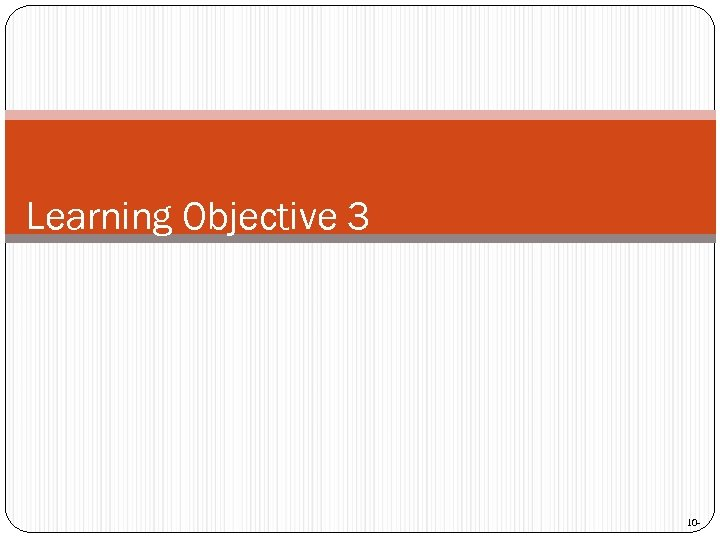Learning Objective 3 10 -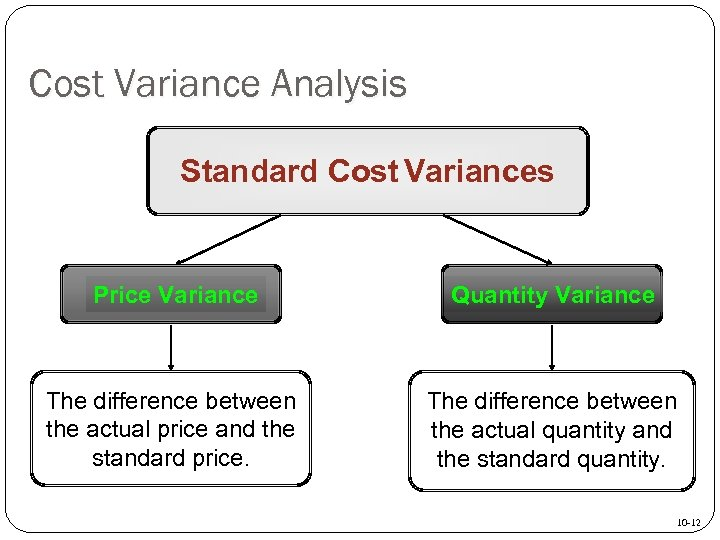Cost Variance Analysis Standard Cost Variances Price Variance Quantity Variance The difference between the actual price and the standard price. The difference between the actual quantity and the standard quantity. 10 -12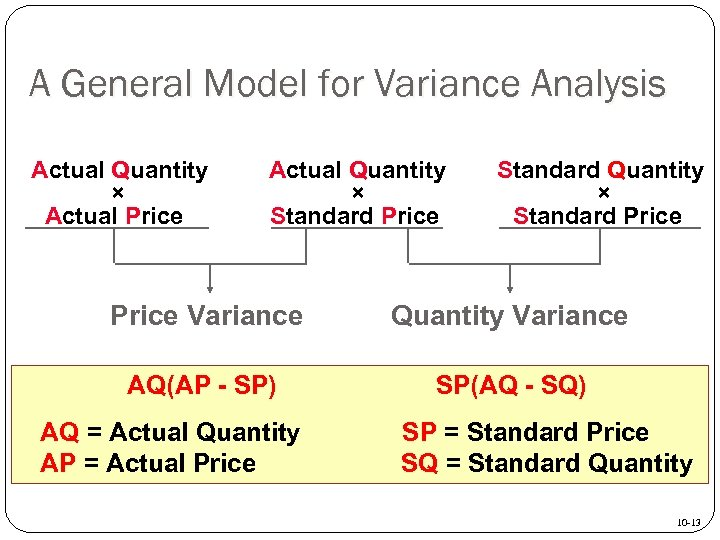A General Model for Variance Analysis Actual Quantity × Actual Price Actual Quantity × Standard Price Variance Materials price SP) AQ(AP - variance Labor rate variance AQ =Variable overhead Actual Quantity AP =spending. Price Actual variance Standard Quantity × Standard Price Quantity Variance Materials quantity variance SP(AQ - SQ) Labor efficiency variance SP = Standard Price Variable overhead SQ = Standard Quantity efficiency variance 10 -13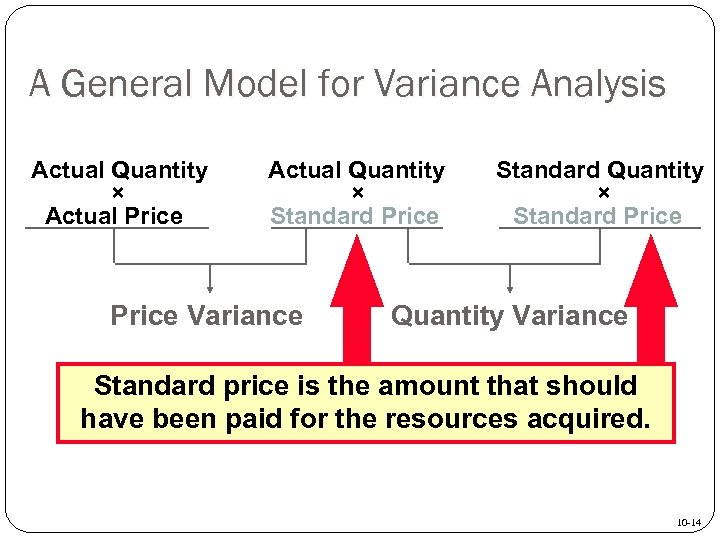A General Model for Variance Analysis Actual Quantity × Actual Price Actual Quantity × Standard Price Variance Standard Quantity × Standard Price Quantity Variance Standard price is the amount that should have been paid for the resources acquired. 10 -14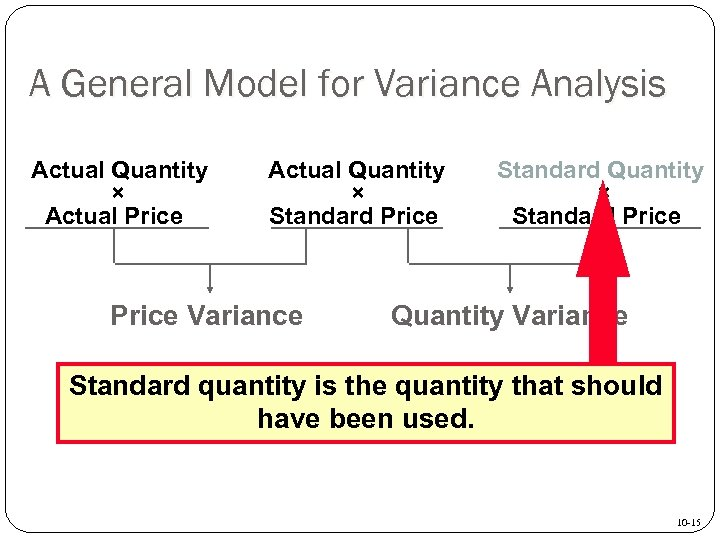A General Model for Variance Analysis Actual Quantity × Actual Price Actual Quantity × Standard Price Variance Standard Quantity × Standard Price Quantity Variance Standard quantity is the quantity that should have been used. 10 -15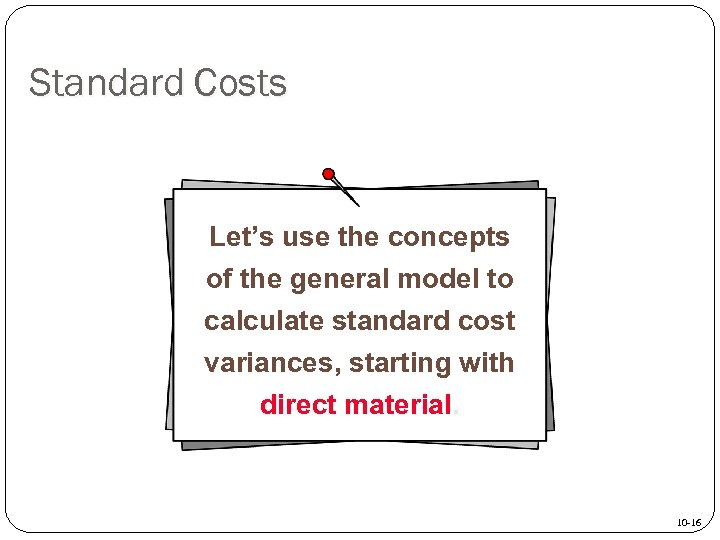Standard Costs Let’s use the concepts of the general model to calculate standard cost variances, starting with direct material. 10 -16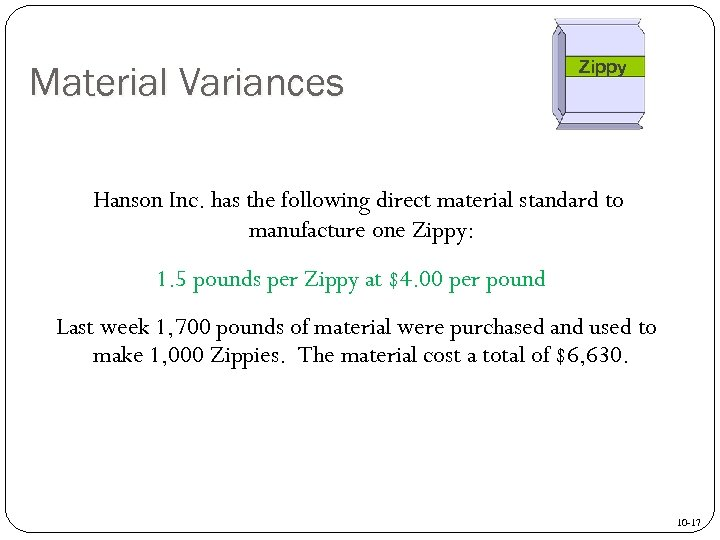Material Variances Zippy Hanson Inc. has the following direct material standard to manufacture one Zippy: 1. 5 pounds per Zippy at \$4. 00 per pound Last week 1, 700 pounds of material were purchased and used to make 1, 000 Zippies. The material cost a total of \$6, 630. 10 -17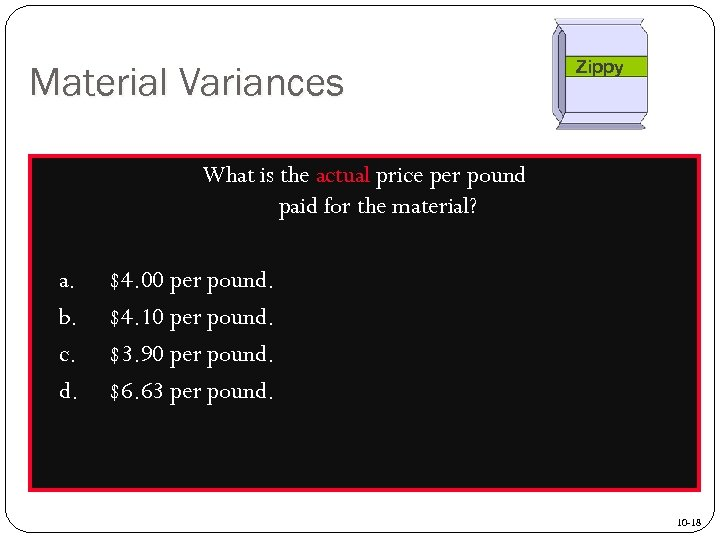Material Variances Zippy What is the actual price per pound paid for the material? a. b. c. d. \$4. 00 per pound. \$4. 10 per pound. \$3. 90 per pound. \$6. 63 per pound. 10 -18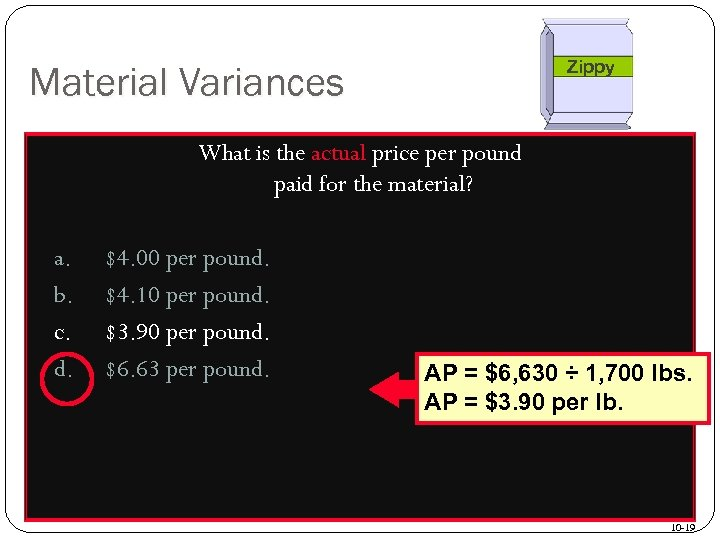Zippy Material Variances What is the actual price per pound paid for the material? a. b. c. d. \$4. 00 per pound. \$4. 10 per pound. \$3. 90 per pound. \$6. 63 per pound. AP = \$6, 630 ÷ 1, 700 lbs. AP = \$3. 90 per lb. 10 -19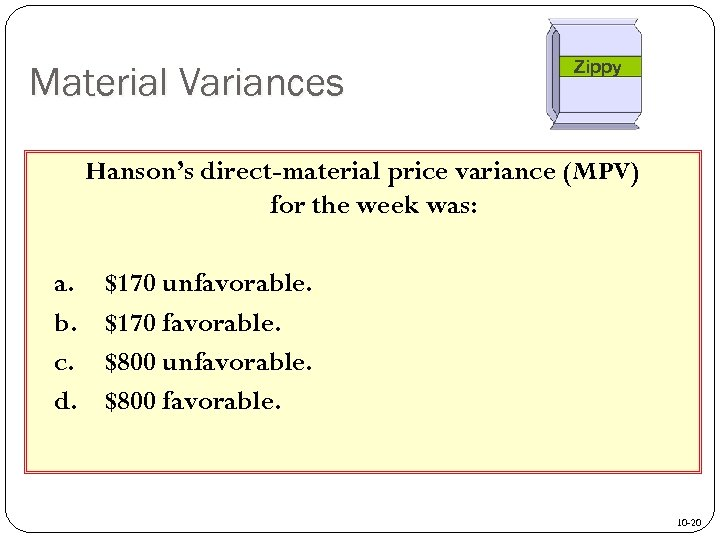Material Variances Zippy Hanson’s direct-material price variance (MPV) for the week was: a. b. c. d. \$170 unfavorable. \$170 favorable. \$800 unfavorable. \$800 favorable. 10 -20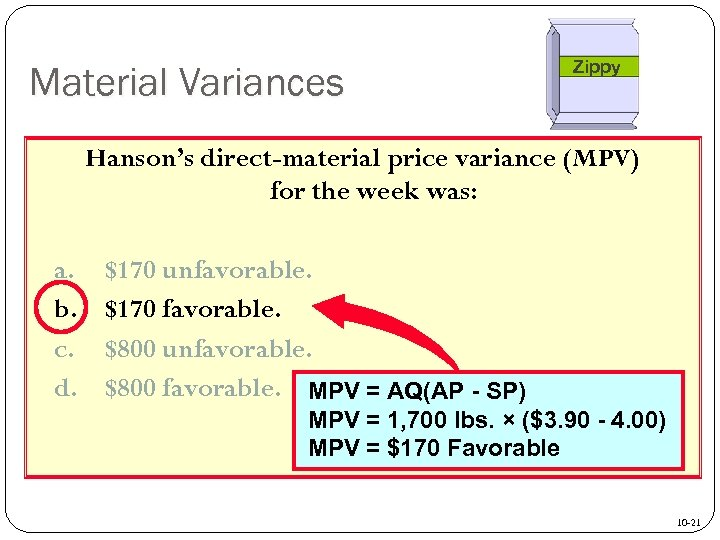Material Variances Zippy Hanson’s direct-material price variance (MPV) for the week was: a. b. c. d. \$170 unfavorable. \$170 favorable. \$800 unfavorable. \$800 favorable. MPV = AQ(AP - SP) MPV = 1, 700 lbs. × (\$3. 90 - 4. 00) MPV = \$170 Favorable 10 -21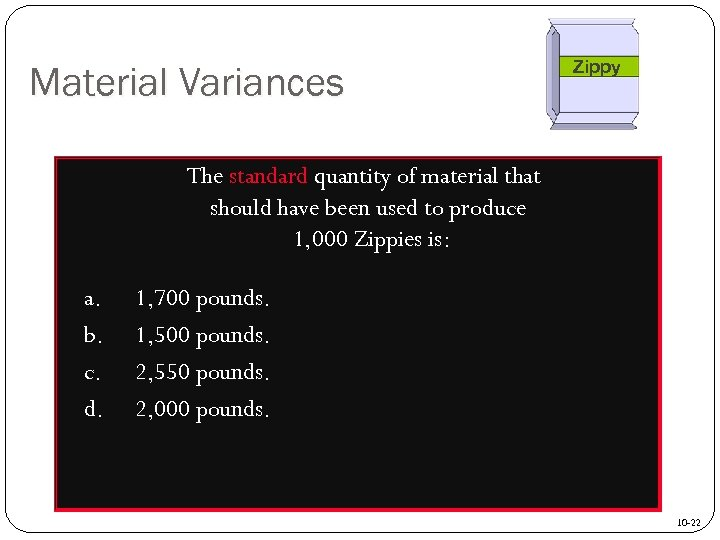Material Variances Zippy The standard quantity of material that should have been used to produce 1, 000 Zippies is: a. b. c. d. 1, 700 pounds. 1, 500 pounds. 2, 550 pounds. 2, 000 pounds. 10 -22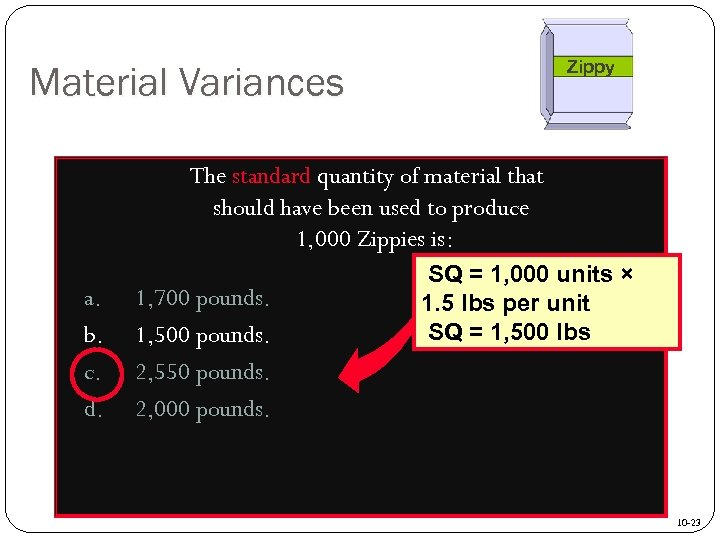Zippy Material Variances The standard quantity of material that should have been used to produce 1, 000 Zippies is: a. b. c. d. 1, 700 pounds. 1, 500 pounds. 2, 550 pounds. 2, 000 pounds. SQ = 1, 000 units × 1. 5 lbs per unit SQ = 1, 500 lbs 10 -23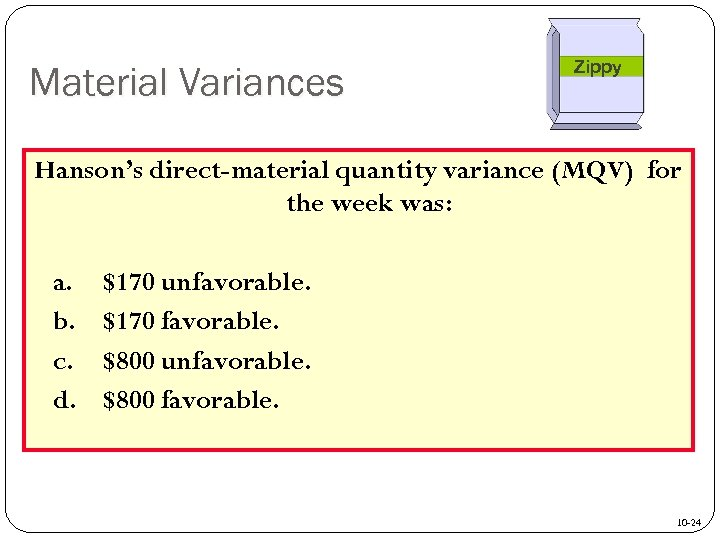Material Variances Zippy Hanson’s direct-material quantity variance (MQV) for the week was: a. b. c. d. \$170 unfavorable. \$170 favorable. \$800 unfavorable. \$800 favorable. 10 -24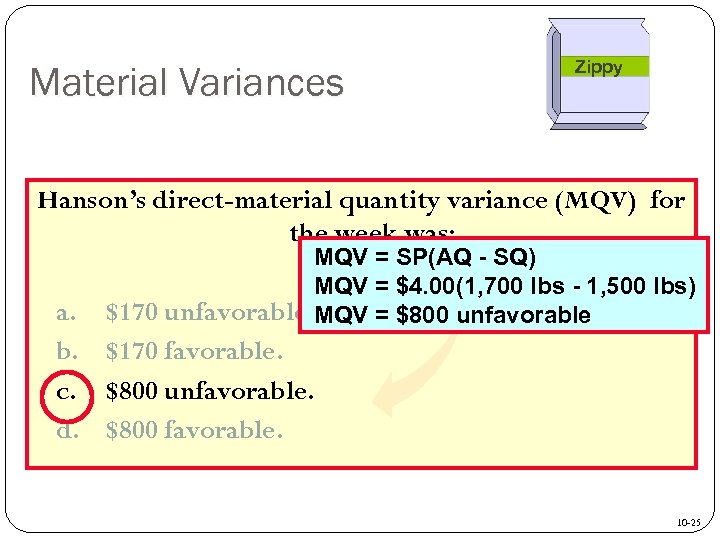Material Variances Zippy Hanson’s direct-material quantity variance (MQV) for the week was: MQV = SP(AQ - SQ) MQV = \$4. 00(1, 700 lbs - 1, 500 lbs) \$170 unfavorable. MQV = \$800 unfavorable a. b. \$170 favorable. c. \$800 unfavorable. d. \$800 favorable. 10 -25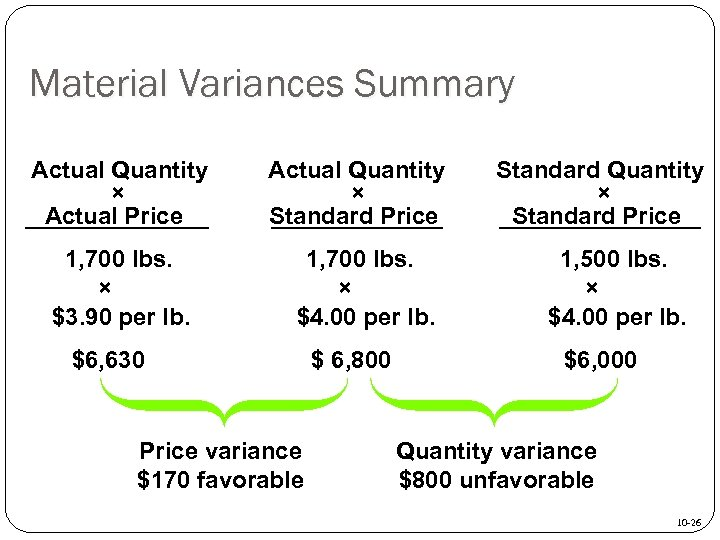Material Variances Summary Actual Quantity × Actual Price 1, 700 lbs. × \$3. 90 per lb. Actual Quantity × Standard Price 1, 700 lbs. × \$4. 00 per lb. \$6, 630 Price variance \$170 favorable \$ 6, 800 Standard Quantity × Standard Price 1, 500 lbs. × \$4. 00 per lb. \$6, 000 Quantity variance \$800 unfavorable 10 -26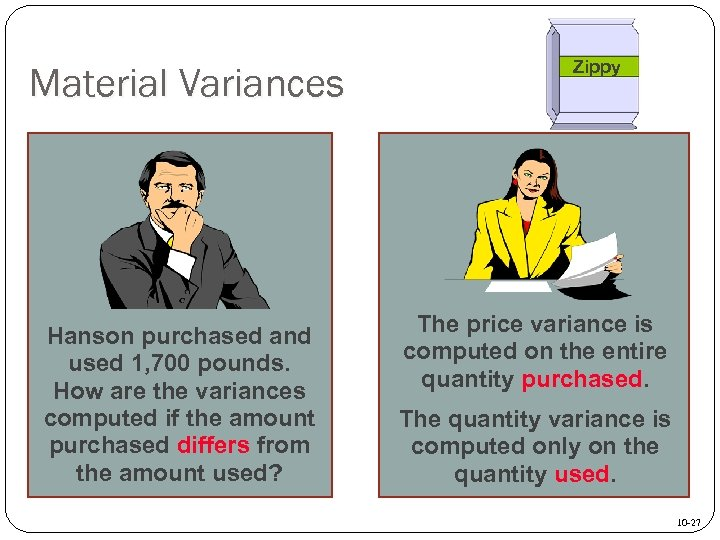Material Variances Hanson purchased and used 1, 700 pounds. How are the variances computed if the amount purchased differs from the amount used? Zippy The price variance is computed on the entire quantity purchased. The quantity variance is computed only on the quantity used. 10 -27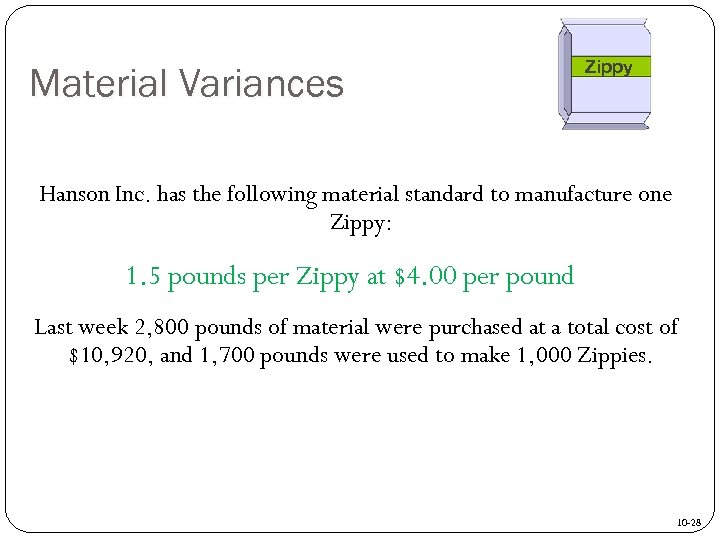Material Variances Zippy Hanson Inc. has the following material standard to manufacture one Zippy: 1. 5 pounds per Zippy at \$4. 00 per pound Last week 2, 800 pounds of material were purchased at a total cost of \$10, 920, and 1, 700 pounds were used to make 1, 000 Zippies. 10 -28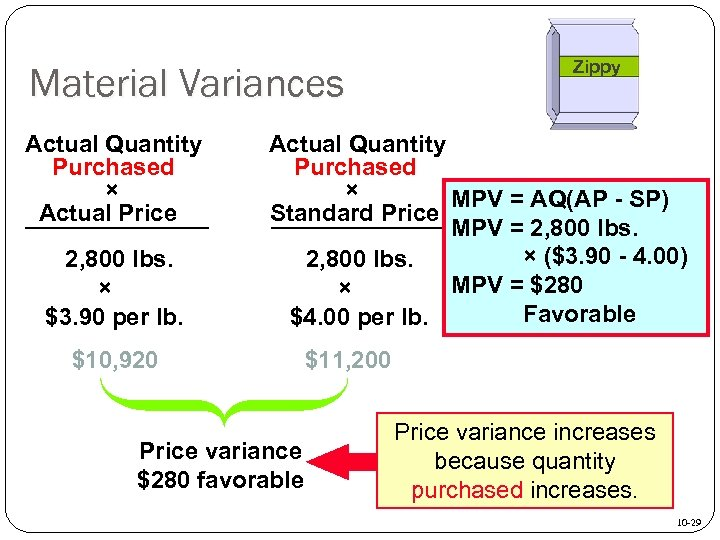Material Variances Actual Quantity Purchased × Actual Price 2, 800 lbs. × \$3. 90 per lb. Zippy Actual Quantity Purchased × MPV = AQ(AP - SP) Standard Price MPV = 2, 800 lbs. × (\$3. 90 - 4. 00) 2, 800 lbs. MPV = \$280 × Favorable \$4. 00 per lb. \$10, 920 Price variance \$280 favorable \$11, 200 Price variance increases because quantity purchased increases. 10 -29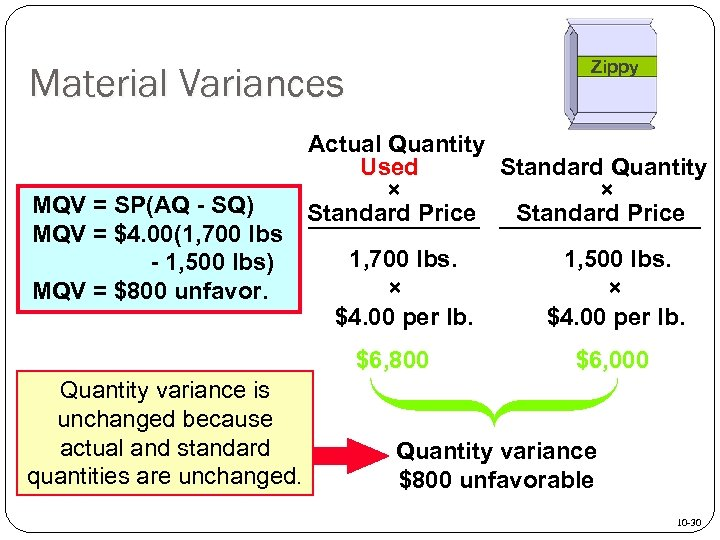Zippy Material Variances MQV = SP(AQ - SQ) MQV = \$4. 00(1, 700 lbs - 1, 500 lbs) MQV = \$800 unfavor. Actual Quantity Used Standard Quantity × × Standard Price 1, 700 lbs. × \$4. 00 per lb. \$6, 800 Quantity variance is unchanged because actual and standard quantities are unchanged. 1, 500 lbs. × \$4. 00 per lb. \$6, 000 Quantity variance \$800 unfavorable 10 -30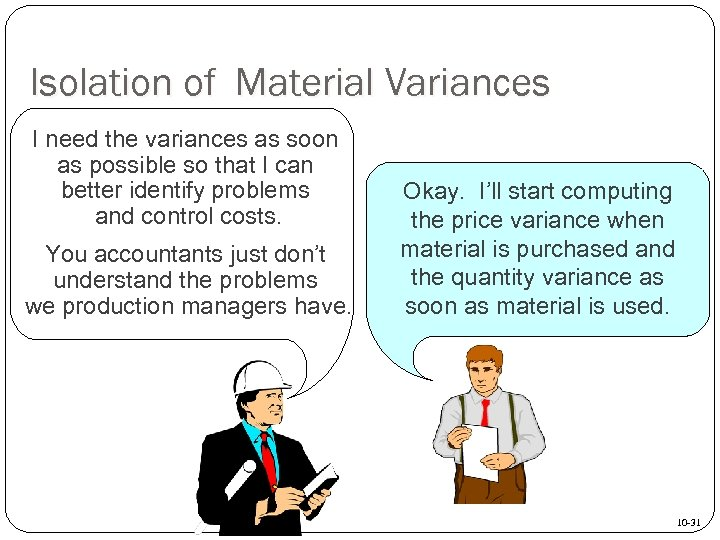Isolation of Material Variances I need the variances as soon as possible so that I can better identify problems and control costs. You accountants just don’t understand the problems we production managers have. Okay. I’ll start computing the price variance when material is purchased and the quantity variance as soon as material is used. 10 -31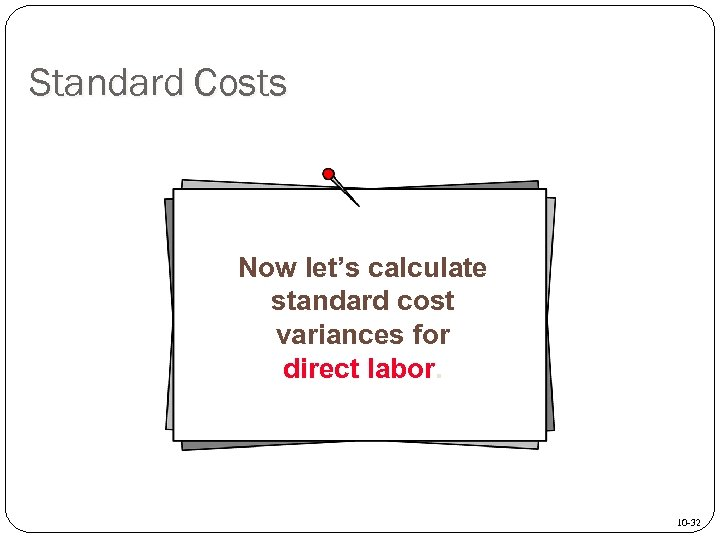Standard Costs Now let’s calculate standard cost variances for direct labor. 10 -32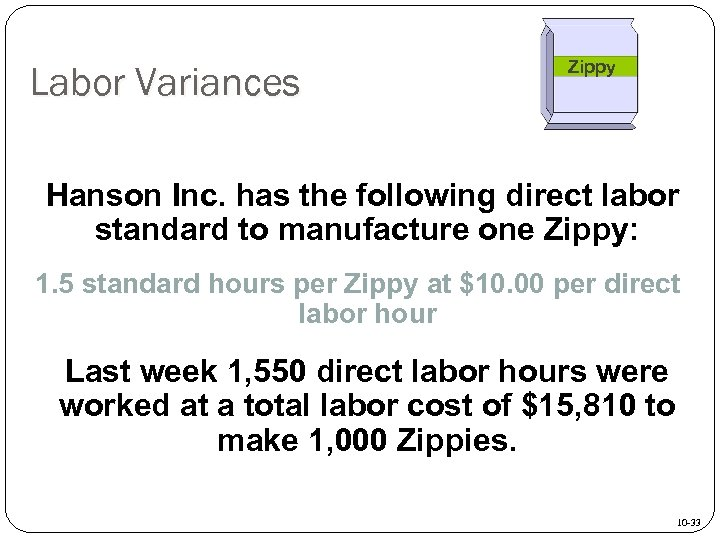Labor Variances Zippy Hanson Inc. has the following direct labor standard to manufacture one Zippy: 1. 5 standard hours per Zippy at \$10. 00 per direct labor hour Last week 1, 550 direct labor hours were worked at a total labor cost of \$15, 810 to make 1, 000 Zippies. 10 -33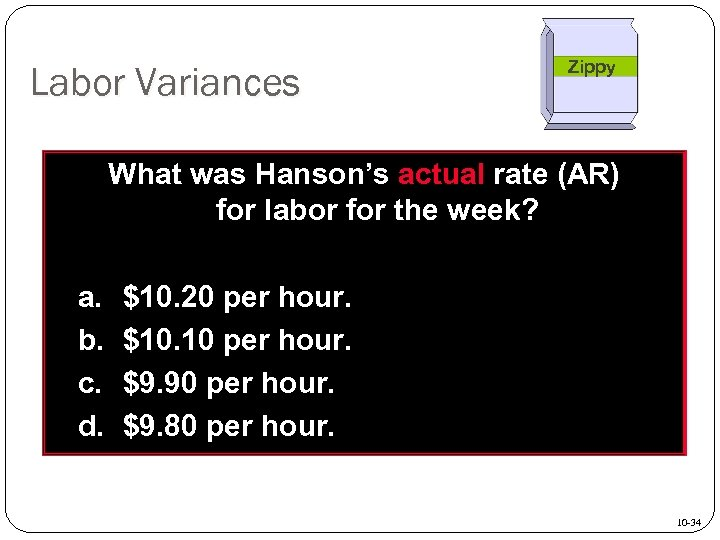Labor Variances Zippy What was Hanson’s actual rate (AR) for labor for the week? a. b. c. d. \$10. 20 per hour. \$10. 10 per hour. \$9. 90 per hour. \$9. 80 per hour. 10 -34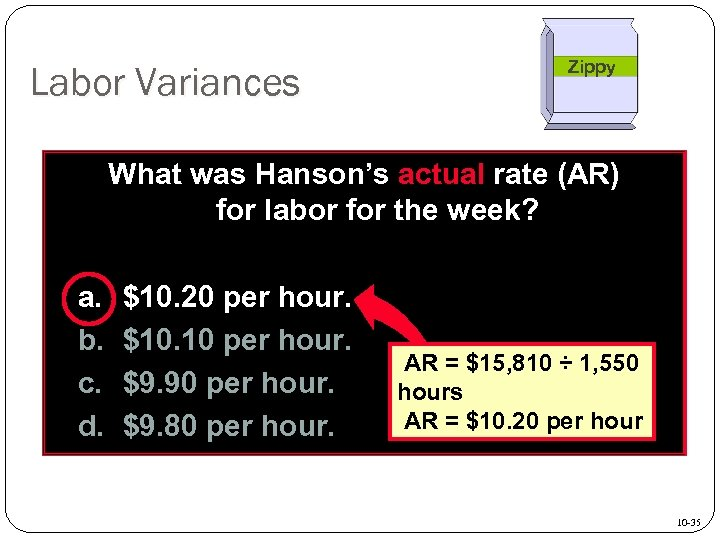Labor Variances Zippy What was Hanson’s actual rate (AR) for labor for the week? a. b. c. d. \$10. 20 per hour. \$10. 10 per hour. \$9. 90 per hour. \$9. 80 per hour. AR = \$15, 810 ÷ 1, 550 hours AR = \$10. 20 per hour 10 -35Labor Variances Zippy Hanson’s labor rate variance (LRV) for the week was: a. b. c. d. \$310 unfavorable. \$310 favorable. \$300 unfavorable. \$300 favorable. 10 -36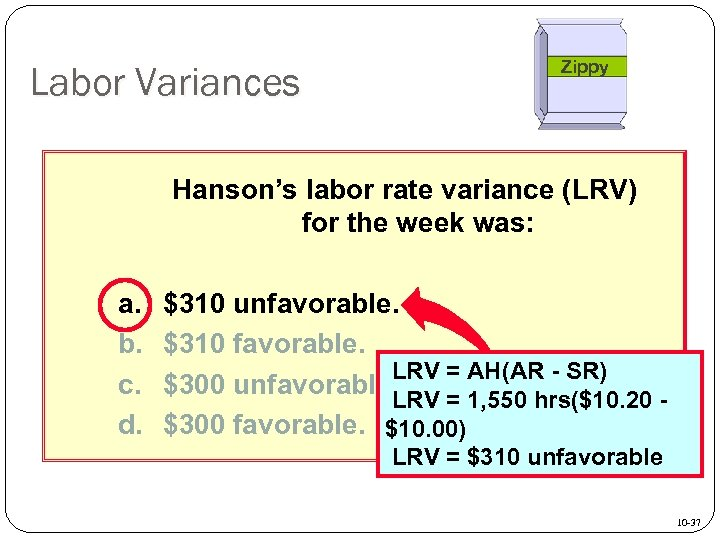Labor Variances Zippy Hanson’s labor rate variance (LRV) for the week was: a. b. c. d. \$310 unfavorable. \$310 favorable. LRV = AH(AR - SR) \$300 unfavorable. LRV = 1, 550 hrs(\$10. 20 \$300 favorable. \$10. 00) LRV = \$310 unfavorable 10 -37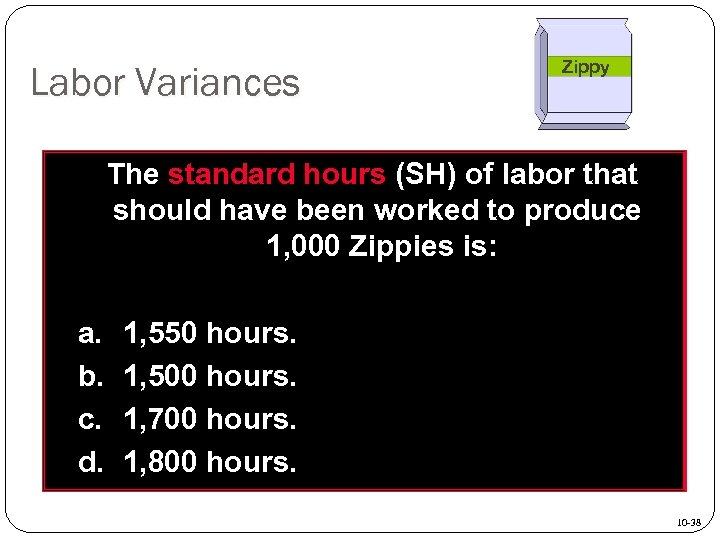Labor Variances Zippy The standard hours (SH) of labor that should have been worked to produce 1, 000 Zippies is: a. b. c. d. 1, 550 hours. 1, 500 hours. 1, 700 hours. 1, 800 hours. 10 -38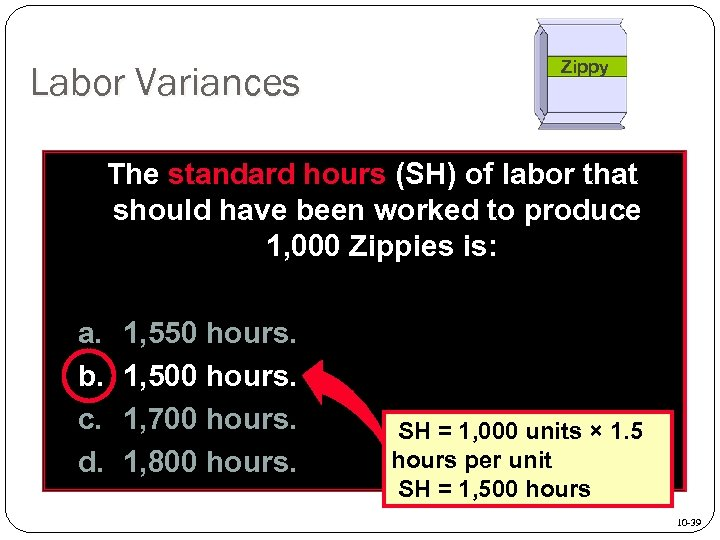Labor Variances Zippy The standard hours (SH) of labor that should have been worked to produce 1, 000 Zippies is: a. b. c. d. 1, 550 hours. 1, 500 hours. 1, 700 hours. 1, 800 hours. SH = 1, 000 units × 1. 5 hours per unit SH = 1, 500 hours 10 -39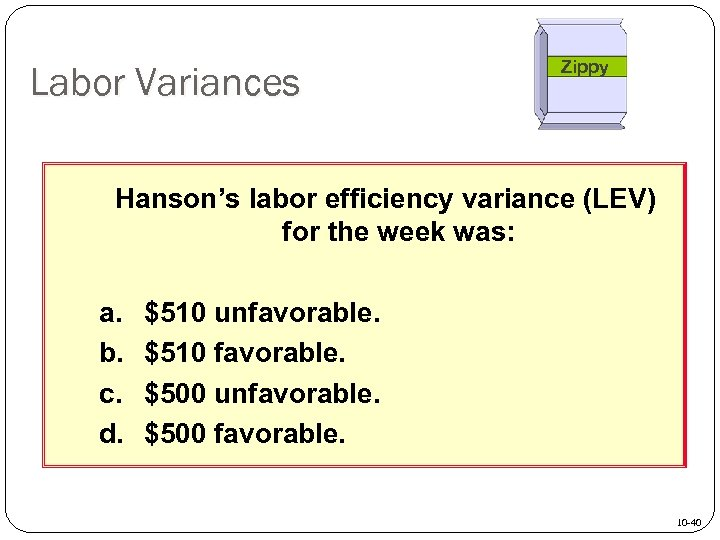Labor Variances Zippy Hanson’s labor efficiency variance (LEV) for the week was: a. b. c. d. \$510 unfavorable. \$510 favorable. \$500 unfavorable. \$500 favorable. 10 -40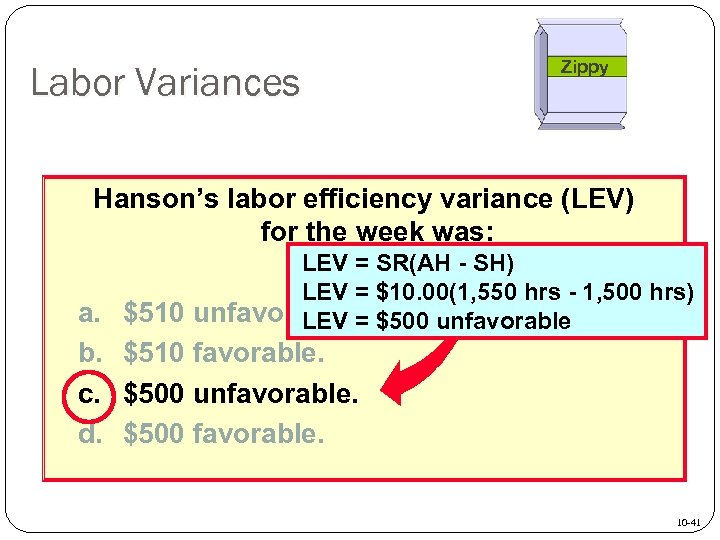Labor Variances Zippy Hanson’s labor efficiency variance (LEV) for the week was: LEV = SR(AH - SH) LEV = \$10. 00(1, 550 hrs - 1, 500 hrs) a. \$510 unfavorable. = \$500 unfavorable LEV b. \$510 favorable. c. \$500 unfavorable. d. \$500 favorable. 10 -41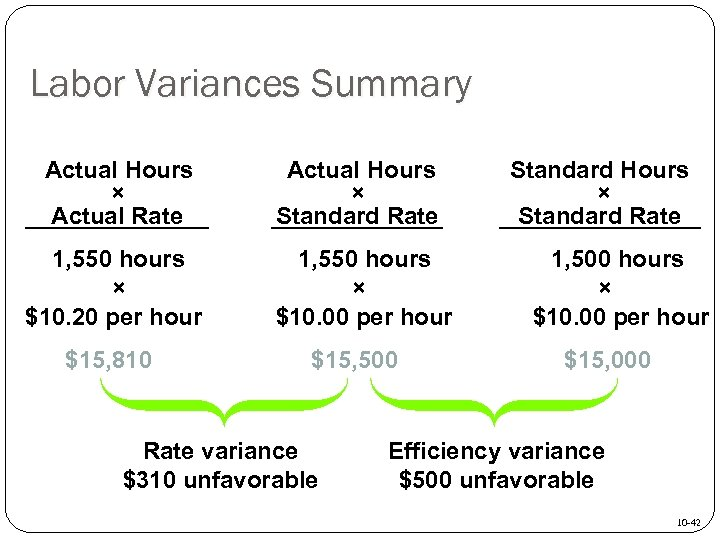Labor Variances Summary Actual Hours × Actual Rate Actual Hours × Standard Rate 1, 550 hours × \$10. 20 per hour 1, 550 hours × \$10. 00 per hour \$15, 810 \$15, 500 Rate variance \$310 unfavorable Standard Hours × Standard Rate 1, 500 hours × \$10. 00 per hour \$15, 000 Efficiency variance \$500 unfavorable 10 -42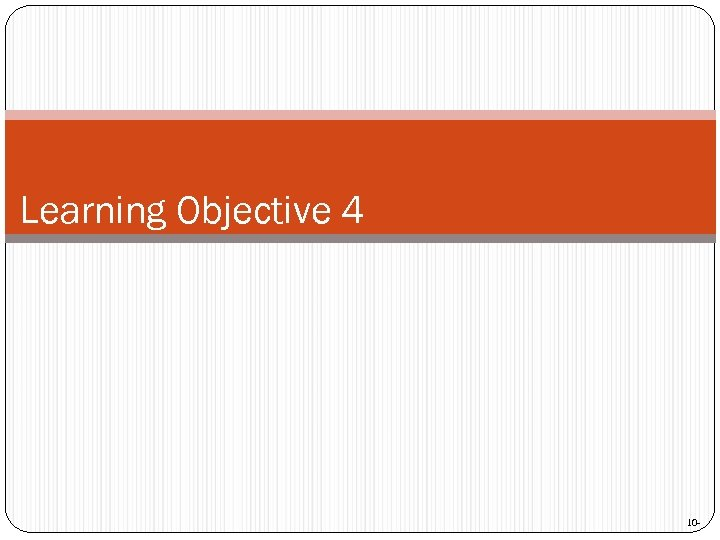Learning Objective 4 10 -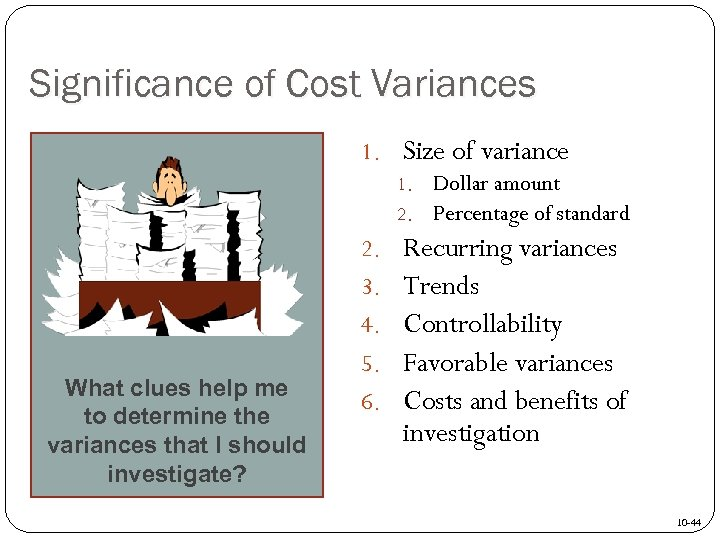Significance of Cost Variances 1. Size of variance 1. Dollar amount 2. Percentage of standard What clues help me to determine the variances that I should investigate? 2. 3. 4. 5. 6. Recurring variances Trends Controllability Favorable variances Costs and benefits of investigation 10 -44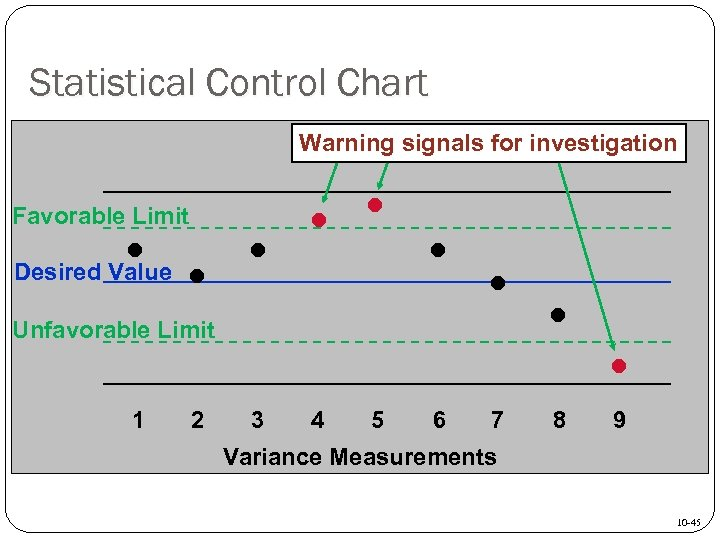Statistical Control Chart Warning signals for investigation Favorable Limit • Desired Value • • • Unfavorable Limit 1 2 3 4 5 6 7 • 8 • 9 Variance Measurements 10 -45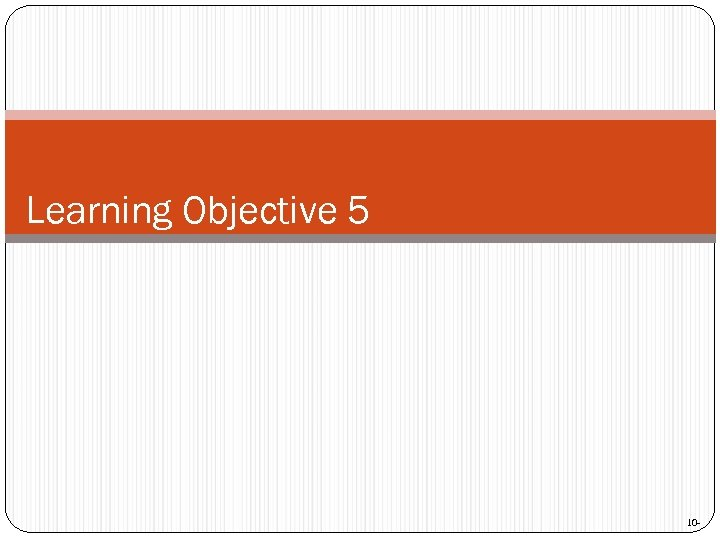Learning Objective 5 10 -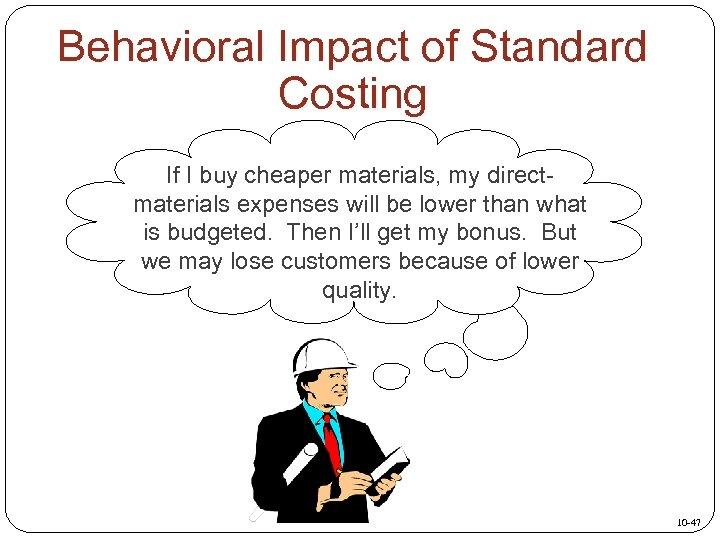Behavioral Impact of Standard Costing If I buy cheaper materials, my directmaterials expenses will be lower than what is budgeted. Then I’ll get my bonus. But we may lose customers because of lower quality. 10 -47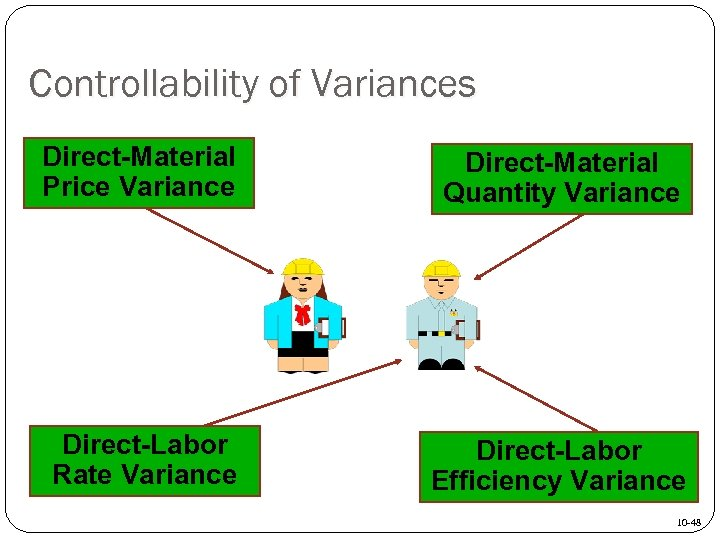Controllability of Variances Direct-Material Price Variance Direct-Material Quantity Variance Direct-Labor Rate Variance Direct-Labor Efficiency Variance 10 -48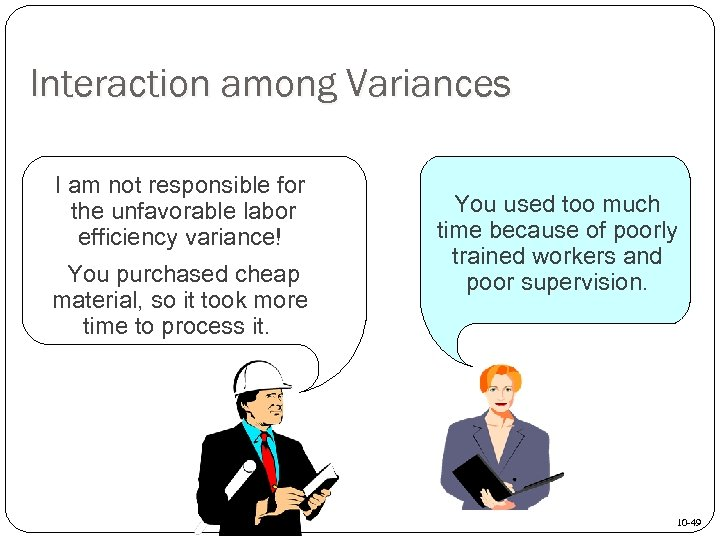Interaction among Variances I am not responsible for the unfavorable labor efficiency variance! You purchased cheap material, so it took more time to process it. You used too much time because of poorly trained workers and poor supervision. 10 -49Learning Objective 6 10 -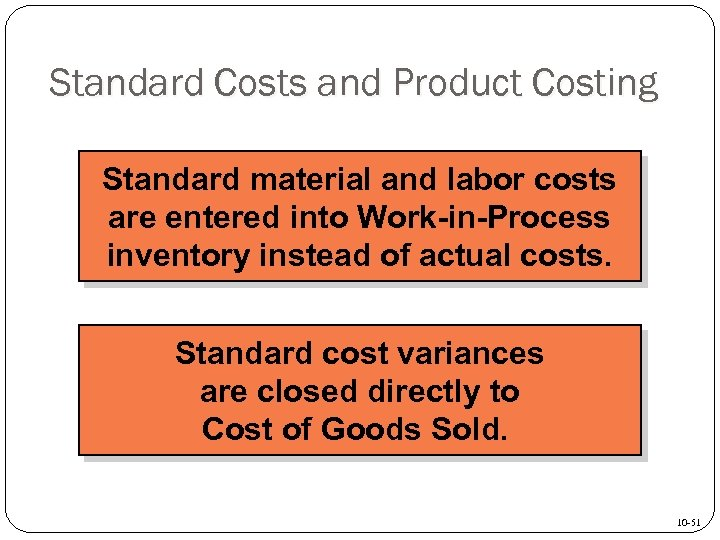Standard Costs and Product Costing Standard material and labor costs are entered into Work-in-Process inventory instead of actual costs. Standard cost variances are closed directly to Cost of Goods Sold. 10 -51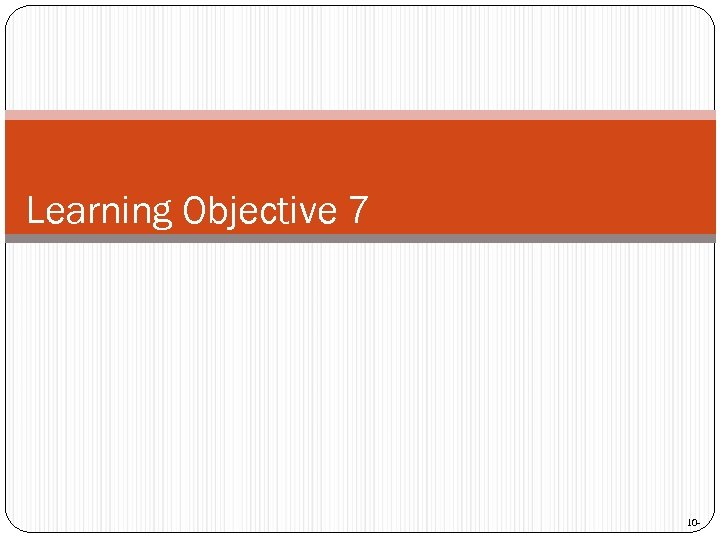Learning Objective 7 10 -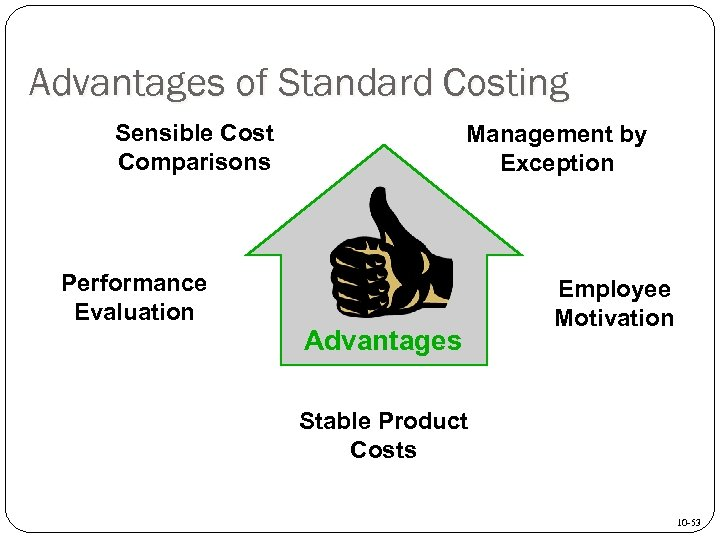Advantages of Standard Costing Sensible Cost Comparisons Performance Evaluation Management by Exception Advantages Employee Motivation Stable Product Costs 10 -53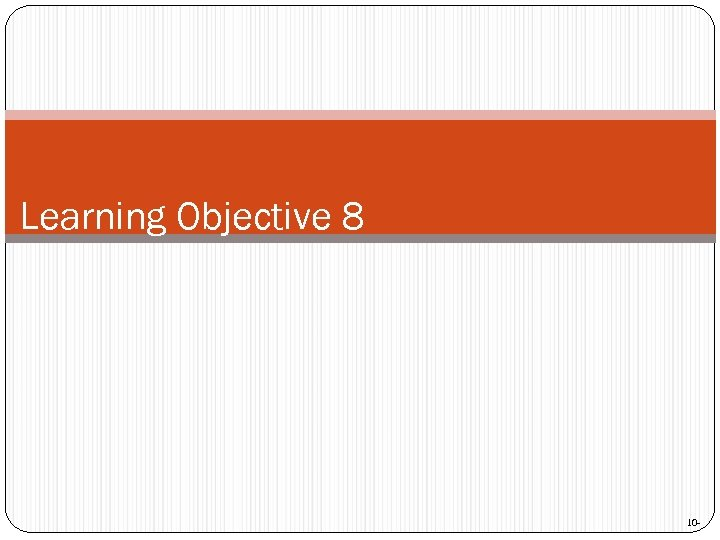Learning Objective 8 10 -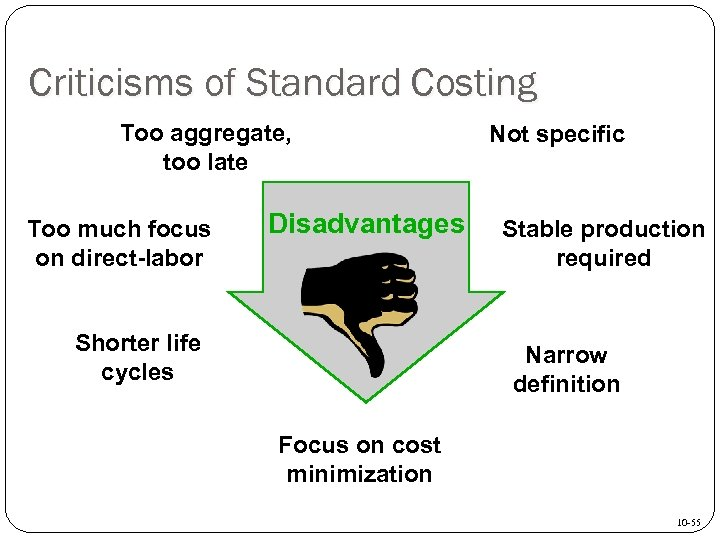Criticisms of Standard Costing Too aggregate, too late Too much focus on direct-labor Disadvantages Shorter life cycles Not specific Stable production required Narrow definition Focus on cost minimization 10 -55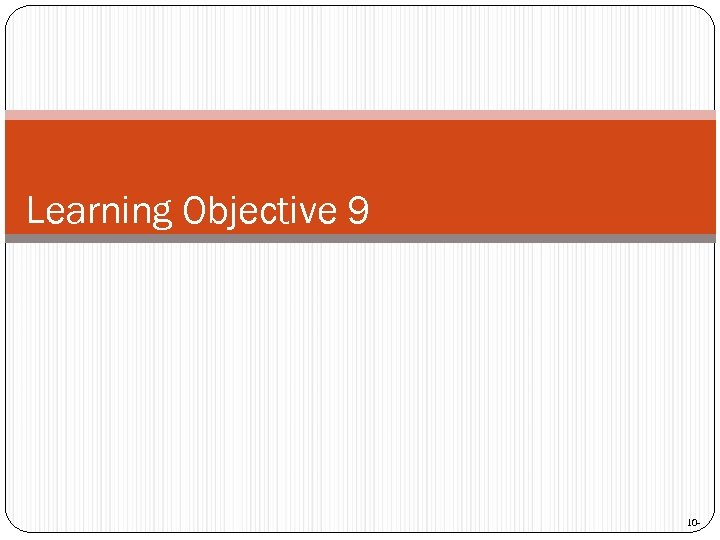Learning Objective 9 10 -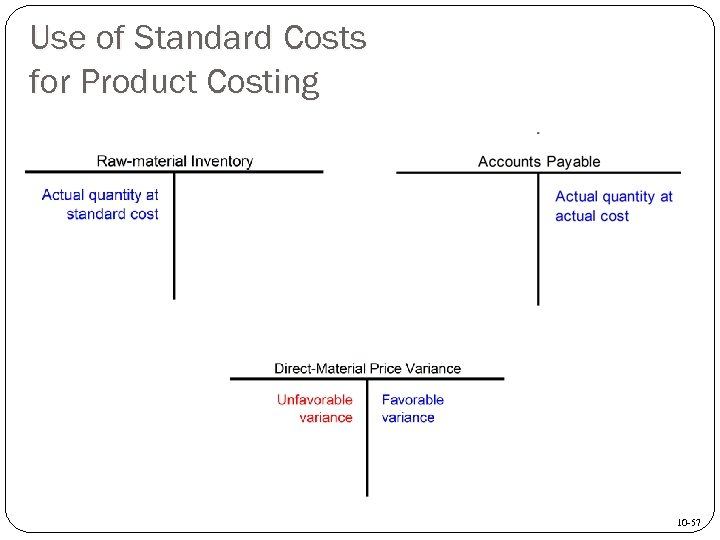Use of Standard Costs for Product Costing 10 -57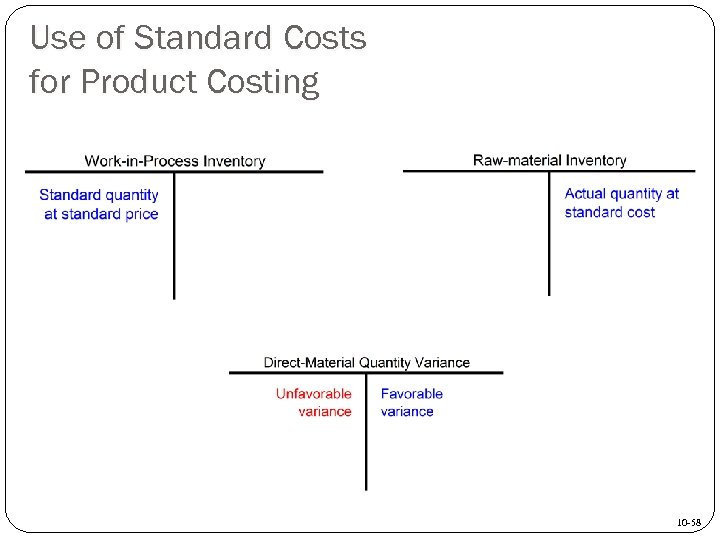Use of Standard Costs for Product Costing 10 -58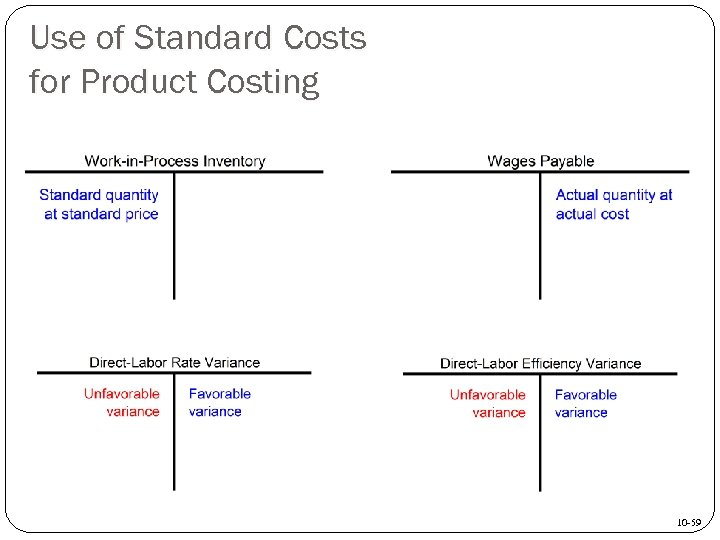Use of Standard Costs for Product Costing 10 -59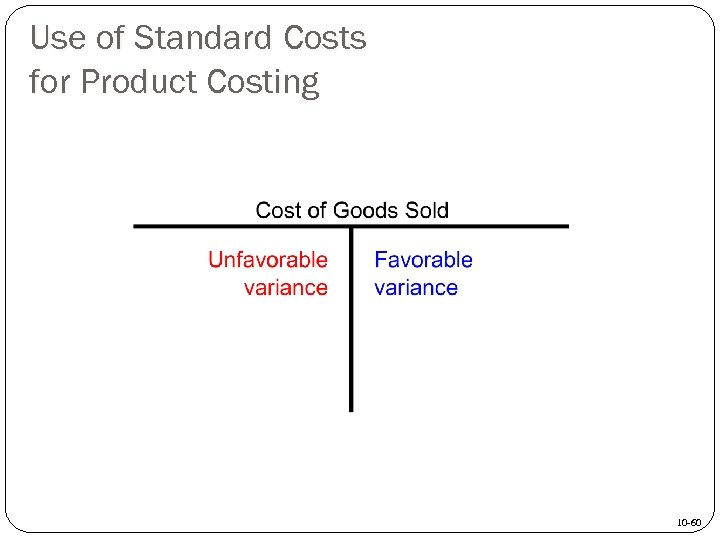Use of Standard Costs for Product Costing 10 -60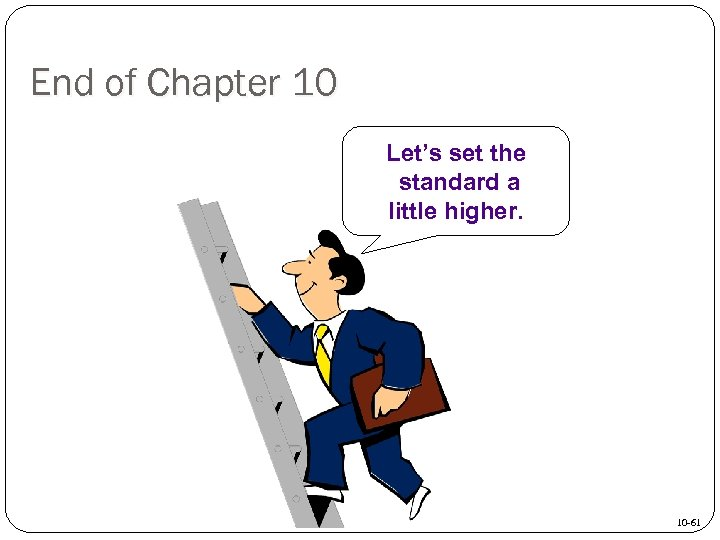End of Chapter 10 Let’s set the standard a little higher. 10 -61